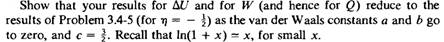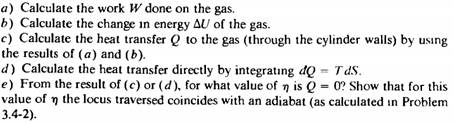### Create an Account

Home / Questions / Repeat parts a b and c of problem 3 4 5 assuming that η 1 2 and that the gas is an i...

# Repeat parts a b and c of problem 3 4 5 assuming that η 1 2 and that the gas is an ideal van der Waals fluid Problem 3 4 5 In a particular engine a gas is compressed in the initial

Repeat parts (a), (b), and (c) of problem 3.4-5, assuming that η = 1/2 and that the gas is an ideal van der Waals fluid.Problem 3.4-5

In a particular engine a gas is compressed in the initial stroke of the piston. Measurements of the instantaneous temperature, carried out during the compression, reveal that the temperature increases accordmg towhere T0 and V0 are the initial temperature and volume, and η is a constant. The gas is compressed to the volume V1 (where V1 0). Assume the gas to be monatomic ideal, and assume the process to be quasi-static.Jun 09 2020 View more View LessSubscribe To Get Solution